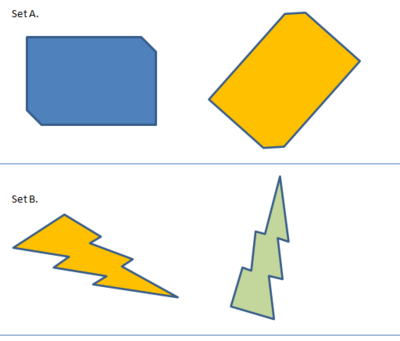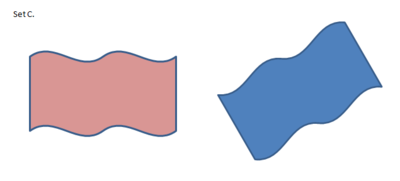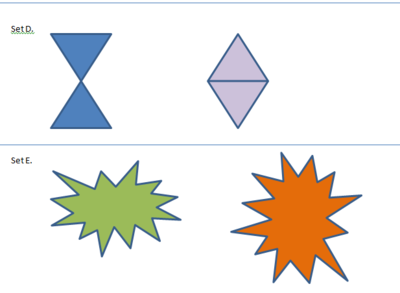# Same Size, Same Shape?

Alignments to Content Standards: 8.G.A

1. For each pair of figures, decide whether these figures are the same size and same shape. Explain your reasoning.2. What does it mean for two figures to be the same size and same shape?

## IM Commentary

The purpose of the task is to help students transition from the informal notion of congruence as "same size, same shape" that they learn in elementary school and begin to develop a definition of congruence in terms of rigid transformations. The task can also be used to illustrate the importance of crafting shared mathematical definitions (MP 6). Note that the term "congruence" is not used in the task; it should be introduced at the end of the discussion as the word we use to capture a more precise meaning of "same size, same shape."

The notion of equivalence is a deep one in mathematics, and in first grade, students begin to investigate what it means for two numbers to be equal (1.OA.D.7). But what does it mean for two geometric figures to be "the same"? In first grade, students begin to study what it means for two one-dimensional figures to have the same length (1.MD.A), in third grade students study what it means for two two-dimensional figures to have the same area (3.MD.C), and in fifth grade they study what it means for two three-dimensional objects to have the same volume (5.MD.C). So by the end of elementary school, students have an idea that the notion of "sameness" is nuanced in a geometric context. They also talk about what it means for two figures to have the same shape, and in every-day language, we say that two rectangles have the "same shape." But what does it mean for two figures to have the "same size and same shape"? If two rectangles have the same area, are they the same size and shape? Without a more precise definition of what we mean by same size, same shape, we can't say yes or no.

A common definition for congruence states that two polygons are congruent if there is a correspondence between the vertices and corresponding sides have the same length and corresponding angles have the same measure. This is fine as far as it goes, but it isn't very useful for talking about the congruence of figures with curved sides. Defining congruence in terms of rigid transformations covers all kinds of figures, and we can show that the traditional definition for polygons follows from it. Since rigid transformations applied to a figure are just a formalization of the idea of picking up that figure and moving it around without stretching or breaking it, this definition also has the advantage of formalizing an intuitive idea of what it means for two figures to be the same size and same shape: if you can move one on top of the other so there are no gaps or overlaps, then they are the same size and same shape.

Used appropriately, this task can initiate a conversation that will lead from the elementary school notion of same size and same shape to the more formal middle school definition of congruence in terms of rigid motions. For the first question, students should have access to a variety of tools, including tracing paper or transparencies, scissors, tape, rulers, protractors etc. After students have worked to articulate a definition of "same size, same shape" either alone or in groups, the class can discuss the merits and drawbacks of the different possible definitions. Teachers should expect students to approach this in at least three different ways:

• Arguments based on the physical appearance of the shape: they look like they are the same size and same shape. If students suggest this type of approach, the teacher can ask whether that means any two rectangles qualify as in some sense they look the same. The same can be asked of circles or ellipses and other non-rectilinear figures. This can help establish that looking similar is not sufficient.
• Arguments based on measurements. Students might measure side lengths and angles and if corresponding sides and angles have the same measurements they could conclude that the two shapes have the same size and shape. The teacher can ask students how they would apply this approach to figures with curved sides, like those in Set C. This can push students toward finding a more general definition.
• Arguments based on superimposing one shape on another and checking that they match up exactly. This can form the basis for the definition of congruence in terms of rigid motions.

Set C raises a very important question about the nature of congruence. If two figures are mirror images, does that "count" as being the same size and shape, or not? (By convention, the mathematical community has agreed that they are, but a different decision could have been made.) Set D raises another question: if a shape has several ''parts,'' can we move them each independently or do we have to move them together? (Again, we agree by convention that the figure must be taken as a whole when defining congruence.)

After a discussion of the possible definitions for "same size, same shape," students who have already been introduced to rigid transformations can then be given the formal definition:

Two figures A and B are congruent if one is the image of the other under a sequence of rigid transformations.

If students have not yet been introduced to rigid transformations, the task can be used to motivate their study. What does it mean to "move one figure on top of another so that they line up exactly?" High school geometry moves in the direction in making these notions of  congruence ("same shape") much more precise, leading one to the careful study of translations, reflections, and rotations. For example, several major milestones in high school geometry involve using these transformations to determine when two triangles are congruent from information about their sides and angles.  In this sense, this task is the start of a transition in which students can come to understand that the notion of equivalence of geometric figures is subtle and deep.

## Solution

a. For set A, these two shapes are rectangles with opposite vertices cut off. They look like they have the same size and the same shape. We can measure the side lengths and angles of the two shapes. If we start at the top left vertex for the blue shape and at the leftmost vertex for the yellow shape, moving around clockwise, the side lengths and angle measurements appear to be the same.

We can also try to align them through rotations, reflections and translations: the top left vertex of the blue shape can be translated over to match up with the leftmost vertex of the yellow shape. We can then rotate the blue shape counterclockwise about this common vertex until it lies perfectly on top of the yellow shape (with sides and vertices matching up).

These two figures appear to be the same size and same shape.

For set B, the approximate size and shape of the two shapes are the same but it is hard to tell definitively from looking (without taking any measurements).  When looking more closely, there is a noticeable difference in the angles. The bottom left vertex of the green shape is approximately equal to a right angle while none of the angles in the yellow shape is close to a right angle. So these two shapes are different.

We can also try to align them through rotations, reflections and translations: the tip of the yellow lightning bolt can be matched up with the tip of the green lightning bolt by a translation. We can then rotate about this common vertex and find that, although they are approximately the same shape, they never match up perfectly, that is either some of the vertices or some of the sides fail to match up.

These two figures do not appear to be the same size and same shape.​

For set C, these two shapes definitely look like they have the same size and shape: they look like two identical flags blowing in the wind and only the color is different. The straight sides of these shapes have the same length. It is not easy to measure the curved sides so measuring doesn't really help us here.

We can also try to align them through rotations, reflections and translations: we can not translate and rotate the red shape to match up with the blue one because the four corners of the two shapes are not identical (for two pairs the angles made at the vertices are acute while for the other two these angles are obtuse). In order to make the two shapes match up, we could move the top right vertex of the red shape to match up with the top right vertex of the blue shape. Then we could rotate the red shape about this common vertex until it shares a common (straight) edge with the blue shape. A reflection about the line containing this edge will then match all parts (angles and sides) up with the blue shape.

These two figures appear to be the same size and same shape.

For set D, each image is a pair of triangles and these triangles look like they have the same size and the same shape. The side lengths of the triangles are all the same and all angles appear to be 60 degrees so these are all equilateral triangles. Each of the first pair of triangles has the same size and the same shape as each of the second pair of triangles. However, the triangles aren't "glued together" in the same way.

We can also try to align them through rotations, reflections and translations: we can translate and rotate each of the individual triangles in the blue pair to match up with one of the triangles from the purple pair. This requires different translations and reflections, however, for each triangle.

These two figures do not appear to be the same size and same shape.​

For Set E, these figures appear to be the same shape but perhaps not the same size--it is difficult to tell without measuring. Careful measuring of side lengths seems to confirm this, and when trying to overlay one on top of the other, they do not match up.

These two figures do not appear to be the same size and same shape.​

b. Two figures are the same size and same shape if one can be moved without stretching or tearing to coincide point-for-point with the other. More formally, two figures are congruent if one is the image of the other under a sequence of rigid transformations.